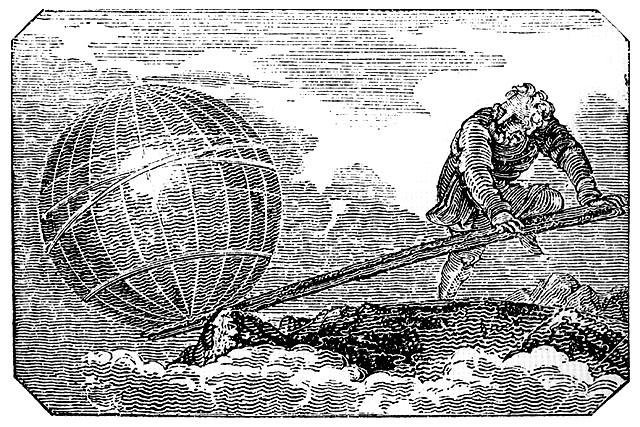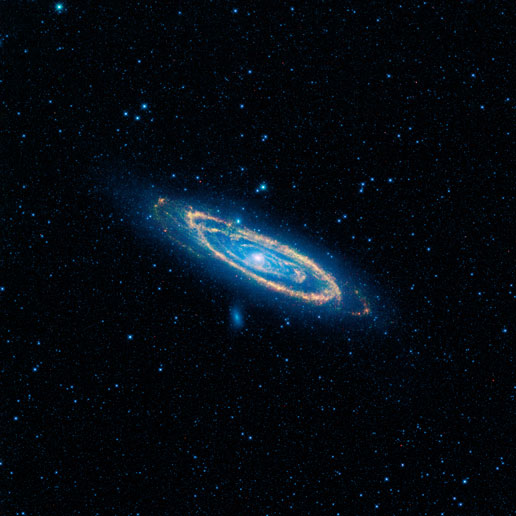Can you move the Earth with a lever?

Updated: October 30, 2013

You all must be familiar with the following, philosophical statement, Give me a place to stand on, and I will move the Earth. Supposedly, this was uttered by Archimedes, and you should all be well familiar with it, and if not, shame on you. Regardless of your moral precondition, though, this is a very good physical question.

Let's assume that you have a lever long and strong enough to try to attempt something like this. Then, let's assume you have a fulcrum placed one meter away from the planet, and then, you want to make some meaningful shift in the planet's position, using the strength of your body alone. Would it work? How would it work? We shall promptly find out.## Some math behind the principle

What Archimedes actually said was, Magnitudes are in equilibrium at distances reciprocally proportional to their weights. Now, in modern reality, this actually has to do with the conservation of energy, and the power applied to one end of the level must be equal to the power exercised on the other end of the level. Therefore, if the fulcrum is placed under the center of the level, the power on both ends must be identical, and for a human to move Earth, this is kind of tricky. However, if you move the fulcrum much closer to the heavier object, you can achieve the desired result with less force, albeit longer movement, so you don't actually conserve on the work you do.

This has a practical appliance in many areas in life. A trivial example would be opening a door. If you apply pressure with your hand further away from the hinges, it is easier to cause the door panel to swing. However, if you apply pressure near the hinges, you will have to exert quite a bit of effort to make the doors swing. Using crowbars is another good example. And so forth. Eventually, it comes down to the ratio between distances from the fulcrum: F(b)/F(a) = A/B.

## Now, the Earth ...

All right, so an average human can probably apply about 75 kgf force on his end of the lever. On the other hand, the Earth can apply 6 x 10^23 kgf. The ratio for lever lengths from the fulcrum is then: 8 x 10^22. Therefore, to create an equilibrium, if we place our planet one meter from the fulcrum, and let's assume that this can be achieved scientifically, then Archimedes would have to sit 8 x 10^22 meters away.

This distance sounds like a lot. Indeed it is. In perfect vacuum, the speed of light is about 300 million meters per second. With 86400 seconds in a given Earth's day, 365 days in a typical orbit around the sun, this translates into roughly 8.45 million light years, more than four times the distance to the nearest galaxy.Now, this only tells us about the distances of the short and long arms of the lever. Now what about the actual displacement? We will have to use the principle of similar triangles here. Plus some extra assumptions. First, we will not dabble into sub-atomic displacements, because that would be just inaccurate. We would have to account for the normal molecular disturbances and whatnot. We need a macroscopic size, so something like one micron perhaps.

In order to move the planet by a meaningful one micron, 10^-6 meters, the human on the far end of our intergalactic level would have to swing a respectable 8.45 light years, or roughly 7.99x 10^13 km. One millimeter would take us a whole millennium just to get going at the pace of human walk. This means our human would have to spend quite a bit of time trying to get the desired result before anything meaningful happened. That's laborious.

## More numbers ...

How much would our lever weigh, you think. If we assume it's made of special stainless steel that does not bend or break beyond the usual human-size lengths, with typical near-room temperature density of about 7.87 grams per cubic centimeter, and the thickness of a typical playground seesaw of 5 cm more or less, then a one meter rod would weigh 15.45 kgf. Sounds about right.

So we have a lever that is roughly 8 x 10^22 meters long, making it weigh 1.24 x 10^24 kgf, which is roughly twice the mass of the planet itself. The gravitation pull of our level would be such that it might get sucked into nearby galaxies, or at least, quite significantly bent. And moving it around might make it collide with other stars or planet, disturbing the stellar matter in a rather naughty way. Or maybe even accrete new matter, growing in size, becoming a stellar pimp.

For those wondering, here's another interesting tidbit. A predicted mathematical neutron star has a mass density of our 4 x 10^17 kg/m^3, or roughly 4 x 10^9 kg/cm^3. Now, with roughly three earth masses balanced on a pinhead, or at least something more reasonable, like a nail, the pressure at the fulcrum would be so significant that we would most likely cause the fusing of protons and electrons, creating a neutron mass, and maybe even precipitating a creation of a black hole, which would then sort of spoil our experiment.

## Conclusion

The answer to the philosophical question is both simple and complex, depends on how you look at it. First, the actually math is quite simple, it's just the inverse ratio between masses and the distances from the fulcrum. Second, making macroscopic displacement in Earth's position using human force requires intergalactic efforts in terms of time and distance. The lever used to move the planet weighs more than the planet itself.

However, we also learn that this kind of experiment would necessitate mechanics beyond human limits, because we would have to use a material than maintains its structural density across millions of light years. Moreover, we would probably be faced with a tricky issue of singularity developing at the fulcrum, causing our experiment to become one gigantic black hole. That would sort of spoil the results somewhat. Here we end happily.

P.S. The images of Archimedes and Andromeda are both in public domain.

Cheers.

You may also like: